### Scribe Post for May 27, 2010

Sunday, May 30, 2010
Hello 9-05. Sorry this is a late scribe ... I honestly forgot ... but this is for the day before the test, if you don't remember.

Anyways, in class we went over Question #21 on Page 403 in the textbook.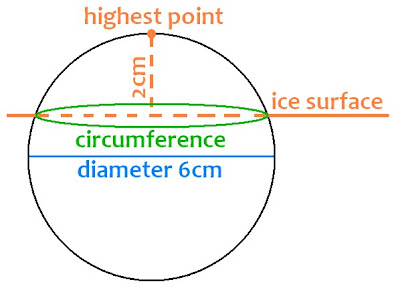*The radius is 3cm because the diameter is 6cm (double the radius)*
Now we need to find out the purple measurement, and since it's a right angle, we could use the "Pythagorean Theorem".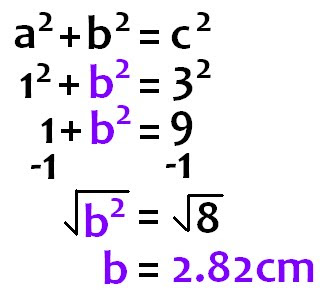Now that we know the radius of the circle that's sticking out of the ice surface (2.82cm) we could find the circumference.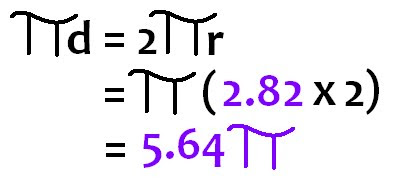The circumference of the circle where the ball touches the ice surface is 5.6(pi).

The rest of the class was work period, and since the test was the next day, Mr.B was asked a lot of questions.

I hope everyone did good on their tests, and good luck on finding out your marks (:
Have fun with what's left of the weekend!
Good luck to all the track people who won't be at school on Monday, and those who will be here, let's have fun again :D

HOMEWORK:
- stashits ---> journal, test signed, 2 problems of the week (including #3)

The next scribe is Marc. I told him on Friday, and reminded him again today. He's doing the test scribe ... but he said he'll do it "laterz" ... so just wait for it (; haha .

### Scribe Post for May 20, 2010

Saturday, May 29, 2010
Hello there, 9-05! Today we started on Chapter 10 which is Circle Geometry. We went straight to 10.1 and started learning or reviewing about circles since I'm sure most of us know something about circles. Chapter 10.1 is about Exploring Angles in a Circle. For this class, we spent our time taking down notes and Mr. B taught us how to find angles.Circumference - The boundary of or distance around a circle.
Diameter - The distance across a circle through its centre.
Radius - A line segment joining the centre of a circle to the outside edge.The angles ABC is called a Central Angle.
Central Angle - An angle form by two radii of a circle.
The straight yellow line from A to C is called a chord.
Chord - A line segment joining two points on the circumference of a circle.Angles ADC and AEC are examples of Subtended Angles.
Subtended Angle: Lying opposite to.
Inscribed Subtended Angle: is equal to half the central triangle.How do you find the measurement from B to C? Use Pythagoras!
First of all, why do we use Pythagoras? We can use Pythagoras method as long as there is a right triangle to find out the missing side length. Do you see the right triangle? If you flip it over or turn your head upside down, you will be able to see a right triangle. So, the angles AC will be represented as the hypotenuse which is c. And let's say that AB represents a and BC represents b.
a^2+b^2 = c^2
6^2+b^2 = 10^2
36+b^2 = 100
b^2 = 100-36
b^2 = 64
Square root b^2 = Square root 64
b = 8
The missing measurement of the line B to C is 8 cm.

Homework:
Chapter 10 Warm-Up #1
10.1 Textbook
-Practice, all odd
-Apply, all even
-Extend and any 2
10.1 Workbook
10.1 Worksheets

Well, that is all for now folks! (: I hope you enjoyed my post! Keep working hard 9-05! For exams are coming soon and we only got about a month of school left! The next scribe is KIM (:
Okay tootles!

### Scribe Post for May 25, 2010

Tuesday, May 25, 2010

Today in math class, we learned about tangents of a circle. First of all, what is a tangent (of a circle)?
A tangent is:
-a line that touches a circle at exactly one point
-the point where the line touches the circle is called the point of tangency

We also learned how to use the tangent chord relationship to find the unknown lengths, given in the diagrams. We used the Pythagorean theorem (only if there's a right triangle).
for example:
-find the length of AB (if BC (diameter) is 14 cm, and AC (hypotenuse) is 20 cm)solution: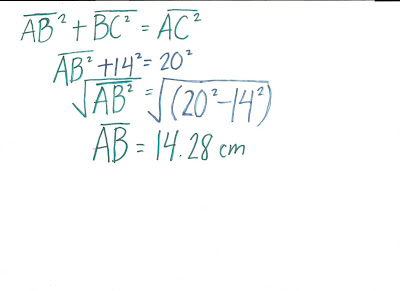Then we learned how to determine angle measures in a circle with a tangent line.
-find the measure of angles A,B,CTo find A
180°=A+42°+90°
180°-132°=A
48°=A

To find C
Since C, is subtended by the same arc as A, A could be divided by two, then you'll get 24° for C.

To find B. (A+B=diameter(which is always 180°)
A+B=180°
48°+B=180°
B=180°-48°
B=132°

After those two examples, we had another example using the tangent chord relationship, once again.
-find the measure of line DEsolution:Today's homework are:
-10.3 textbook
-CYU #2
-Practice (odd)
-Apply : ALL
-Extend 19&21
-Workbook, and Extra Practice
-journal
TEST ON FRIDAY

For Tuesday, June 1
hand in inside stash-its
-problems of the week (#3, and another)
-journal
-last test signed

### Scribe May 21

Monday, May 24, 2010
SCRIBE

Um hello... in class on Friday we learned more things about circles. Chord Properties to be exact. There are four steps to find the interscection of the arcs... Heres a summary of the things we leanred in class recently.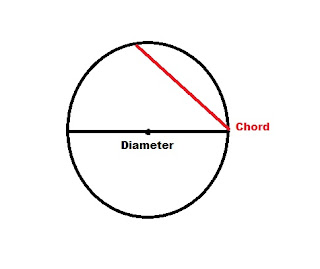1) You make a chord on the circle.2)Widen your compass pass ther half way point of your line.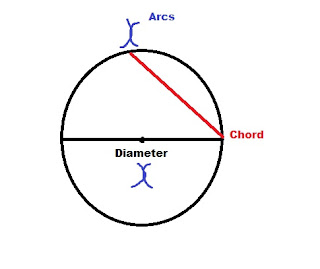3)Place compass point on each end of the line. Make arcs above and below the line.

4) Then draw lines through the intersection of the arcs and the center point of the circle.( sorry the line that intersects the arcs were suppose to be straight not slanted like this...)

Then heres an example of a question we did, based on the circle we have drawn. We were given that the Chored is 2x and we needed to find the radius of the circle.

Then after he gave us another question. We had to find the length of CD if CB is 2x+2. To do that we need to do pythagras and use the information he gave us. if CB is 2x+2 and AD which is equal to BD then that means the side is x.
Here's how you solve it.

Well... this is it... the homework is 10.2.
extra practise
Workbook
mental Math
In the workbook its...
CYU 1 and 2 (pg.389)
Practise and 2
Apply odd
Extend all

### Scribe post for May 11

Thursday, May 13, 2010
Hello 905. Sorry about the lack of scribe posts. So here's for the class two days ago.

Today in class we started Chapter 9. * Inequalities*

So we started up with some symbols we knew, and some we didn't know.Here are some examples of how we use these symbols. (algebraically)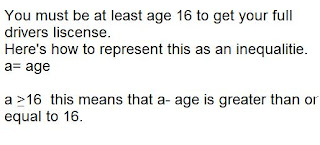And here is how you express it graphically.2- step Inequalities.

How do you solve this?

just like in linear equations.Ok theres the quick overview of what we did in class. Sorry if I missed some things, I got the main points down I think. Homework was
9.1 All odd, or even numbers up to 22.
Extend 23+ 24 or 23+ 25

And today for homework.
Practice all odd numbers.
All of apply
Extend 23 and 26
Choose two of 24, 25, 27, 28
Then next person for scribe would be Melissa. Please comment.

### Scribe Post for April 29, 2010

Sunday, May 2, 2010
Hey everyone! On Thursday April 29, we learned about the term "Slope."

m = slope

y =[mx] + [b] y intercept
slope ^

Slope formula:
y2 - y1 = m
x2 - x1

Ex. (0.1, o.023)
(0.4, 0.092)

y2 - y1 = 0.092 - 0.023
x2 - x1 0.4 - 0.1

= 0.069
0.3

= 0.23

y intercept formula:
y - y1 = m (x-x)

Ex. (0.1, 0.023) (0.4, 0.092)
(x1, y1) (x2, y2)

y - y1 = m (x-x)
y - 0.023 = 0.23 (x-0.1)
y - 0.023 = 0.23x -0.023
y = 0.23x - 0.023 + 0.023
y = 0.23x

HOMEWORK!
Review
The test
Rest of the sheets!

### Scribe Post for April 28, 2010

Wednesday, April 28, 2010
Hello 9-05! Today in class we were given 3 problems. These problems were hard for some people so I will explain it to you!

1) A bag contains 36 marbles. There are one quarter as many purple marbles as red ones. There are three times as many green as purple marbles. How many green marbles in the bag?

There are 36 marbles in the bag.
so Red + Purple + Green = 36
We don't know how many red marbles there are so red will be x. There is 1/4 purple marbles out of the red ones so purple will be 1/4x. And lastly, for green, there are 3 times the number of purple marbles so green will represent 3(1/4x).

So you have to add all of those to get the number of marble in the bag.
x + 1/4x + 3(1/4x) = 36
That is your equation. Now solve for x.

So there are 18 red marbles. Now you fill in x will 18 to find out how many green marbles there are in the bag.

3(1/4x) = Number of green marbles
3(18/4) = Green
3(4.5) = Green
13.5 = green

There are 13.5 green marbles in the bag.

2) A skier takes 1.2 minutes to complete a run of 2 km. How fast in meters/second was he going?

First you must convert minutes into seconds and kilometers into meters. There are 60 sec in 1 min and there are 1000 meters in 1 km.

1.2(60)=72 sec
2(1000)=2000 m

Then you use to formula s=d/t.

He was going at a speed of 27.78 m/s.

3) A regular hexagon has 6 sides. What is the measure of one interior angle? What is the measure of all interior angles?

There are 6 triangles in a hexagon.

In an equilateral triangle the interior angles are 60 degrees.

You add them to get the interior angle of a hexagon.

60+60=120 degrees

One interior angle of a hexagon is 120 degrees.
To find them all out you multiply one angle by 6.

120(6)=720 degrees
All of the interior angles are 720 degrees.

Homework:
8.4 Text Book Work
-1-23 and 26-30
White Sheets
-All up to Age Problems